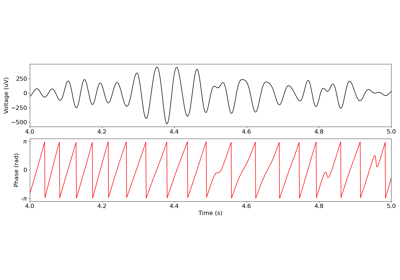# neurodsp.timefrequency.phase_by_time¶

neurodsp.timefrequency.phase_by_time(sig, fs, f_range=None, hilbert_increase_n=False, remove_edges=True, **filter_kwargs)[source]

Compute the instantaneous phase of a time series.

Parameters
sig1d array

Time series.

fsfloat

Sampling rate, in Hz.

f_rangetuple of float or None, optional default: None

Filter range, in Hz, as (low, high). If None, no filtering is applied.

hilbert_increase_nbool, optional, default: False

If True, zero pad the signal’s length to the next power of 2 for the Hilbert transform. This is because scipy.signal.hilbert can be very slow for some lengths of x.

remove_edgesbool, optional, default: True

If True, replace samples that are within half of the filter’s length to the edge with nan. This removes edge artifacts from the filtered signal. Only used if f_range is defined.

**filter_kwargs

Keyword parameters to pass to filter_signal.

Returns
pha1d array

Instantaneous phase time series.

Examples

Compute the instantaneous phase, for the alpha range:

>>> from neurodsp.sim import sim_combined
>>> sig = sim_combined(n_seconds=10, fs=500,
...                    components={'sim_powerlaw': {}, 'sim_oscillation': {'freq': 10}})
>>> pha = phase_by_time(sig, fs=500, f_range=(8, 12))


## Examples using neurodsp.timefrequency.phase_by_time¶